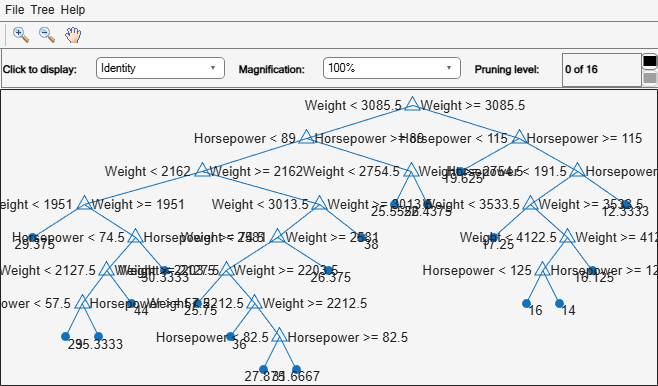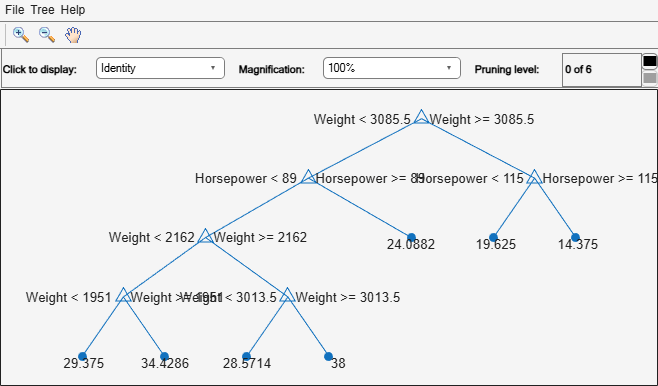# prune

Produce sequence of regression subtrees by pruning

## Syntax

``tree1 = prune(tree)``
``tree1 = prune(tree,Name=Value)``

## Description

````tree1 = prune(tree)` creates a copy of the regression tree `tree` with its optimal pruning sequence filled in.```

example

````tree1 = prune(tree,Name=Value)` creates a pruned tree with additional options specified by a single name-value argument.```

## Examples

collapse all

Load the `carsmall` data set. Consider `Horsepower` and `Weight` as predictor variables.

```load carsmall; X = [Weight Horsepower]; varNames = ["Weight" "Horsepower"];```

Grow a regression tree using the entire data set. View the tree.

`Mdl = fitrtree(X,MPG,PredictorNames=varNames)`
```Mdl = RegressionTree PredictorNames: {'Weight' 'Horsepower'} ResponseName: 'Y' CategoricalPredictors: [] ResponseTransform: 'none' NumObservations: 94 ```
`view(Mdl,Mode="graph");`The regression tree has 16 pruning levels.

Prune the regression tree to pruning-level 10. View the pruned tree.

```MdlPruned = prune(Mdl,Level=10); view(MdlPruned,Mode="graph");```The pruned tree has six pruning levels.

## Input Arguments

collapse all

Regression tree, specified as a `RegressionTree` object created using the `fitrtree` function.

### Name-Value Arguments

Specify optional pairs of arguments as `Name1=Value1,...,NameN=ValueN`, where `Name` is the argument name and `Value` is the corresponding value. Name-value arguments must appear after other arguments, but the order of the pairs does not matter.

Example: `tree1 = prune(tree,Level=10)` prunes the tree to level ten.

Before R2021a, use commas to separate each name and value, and enclose `Name` in quotes.

Example: `tree1 = prune(tree,"Level",10)` prunes the tree to level ten.

Pruning cost, specified as a numeric scalar from `0` (no pruning) to `1` (prune to one node). The `prune` function prunes the tree to minimize the sum of (`Alpha` times the number of leaf nodes) and a cost (mean squared error).

Use only one of these three name-value arguments at a time: `Alpha`, `Level`, or `Nodes`.

Pruning level, specified as a numeric scalar from `0` (no pruning) to the largest pruning level of this tree `max(tree.PruneList)`. The `prune` function returns the tree pruned to this level.

Use only one of these three name-value arguments at a time: `Alpha`, `Level`, or `Nodes`.

Branch nodes to turn into leaf nodes, specified as a numeric vector with elements from `1` to `tree.NumNodes`. Any `tree` branch nodes listed in `Nodes` become leaf nodes in `tree1`, unless their parent nodes are also pruned.

Use only one of these three name-value arguments at a time: `Alpha`, `Level`, or `Nodes`.

## Output Arguments

collapse all

Pruned regression tree, returned as a `RegressionTree` object.

## Tips

• `tree1 = prune(tree)` returns the decision tree `tree1` that is the full, unpruned `tree`, but with optimal pruning information added. This is useful only if you created `tree` by pruning another tree, or by using `fitrtree` with pruning set `"off"`. If you plan to prune a tree multiple times along the optimal pruning sequence, it is more efficient to create the optimal pruning sequence first.

## Version History

Introduced in R2011a